### Creating equations

Equation Editor lets you insert preset equations or you can also build your own equation by inserting preset templates and symbols.

Symbols are single characters, such as logic symbols and Greek characters. A template consists of a symbol and, in some cases, writable areas, or slots, for adding variables or other elements. For example, a fraction template provides two slots, one for the numerator and one for the denominator, that are separated by a line (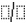). You can insert these equation elements by choosing them in Equation Editor or by using shortcut keys.

Each equation you insert into a document is treated as one item rather than individual symbols or characters. The equation is inserted into a document inside a graphics box, which you can move, size, and edit. For more information about formatting a graphics box, see “Inserting graphics boxes and text boxes.”

#### To insert a preset equation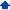1 Click where you want to insert an equation.
 2 Click Insert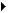Equation.
 3 Click the Edit equation tab.
 4 Click Presets.
 5 In the Equation presets dialog box, choose an equation from the Choose a preset list.

#### To insert a symbol into an equation by using a shortcut key1 Select the equation in which you want to insert a symbol.
 2 Click Edit(Open) equation object.
 3 Click where you want to insert a symbol.

 To insert Press Ctrl + K, and press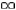I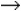A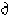D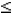< or Shift + <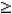> or Shift + >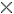T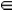E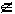Shift + E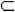C15" border="0" hspace="0" vspace="0"> Shift + C

The following shortcut keys attach the indicated mark to the character to the left of the cursor.

 To apply Press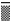Ctrl + Shift + Hyphen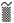Ctrl + Shift + ~ (Ctrl + Shift + “ on some keyboards)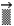Ctrl + Alt + - (hyphen)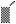Ctrl + Alt + ‘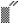Ctrl + Shift + “ (Ctrl + Shift + ~ on some keyboards)Ctrl + Alt+ .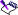• In Equation Editor, the Spacebar is disabled unless the active style is Other. However, when you apply Standard or Greek styles, you can insert spaces into an equation by using shortcut keys. For information about styles, see “Formatting equation elements.”

#### To insert an equation template by using a shortcut key1 Click where you want to insert an equation template into a document.
 2 Click InsertEquation.
 3 Click the Edit equation tab.

 To insert Press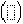Ctrl + ) or Ctrl + (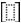Ctrl + ] or Ctrl + [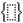Ctrl + Shift + } or Ctrl + Shift + {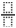Ctrl + FCtrl + /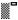Ctrl + H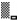Ctrl + L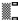Ctrl + JCtrl + I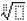Ctrl + T, then N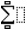Ctrl + T, then S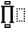Ctrl + T, then P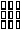Ctrl + T, then M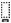Ctrl + T, then U• Keyboard shortcuts such as Ctrl + T, then N indicate a two-step process; that is, first press Ctrl and T, release those keys, and then press N.

#### To insert a space in an equation by using a shortcut key1 Select the equation in which you want to insert a space.
 2 Click Edit(Open) equation object.
 3 Click in the equation where you want to insert a space.

 To insert Press a zero space Shift + Spacebar a thin space Ctrl + Alt + Spacebar a figure space Ctrl + Spacebar an em space Ctrl + Shift + Spacebar